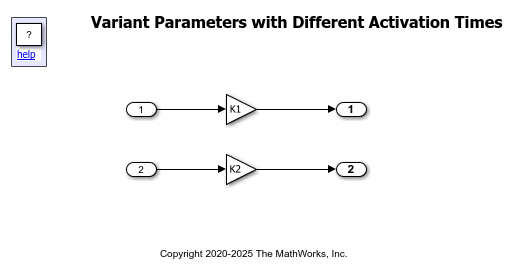# Create a Simple Variant Parameter Model

This example shows you how to create variant parameters and use them in Simulink® blocks to vary the block parameter values based on variant conditions.

Variant parameters give you a way to represent block parameters that can have multiple choice values. For example, you may implement a system where the functional behavior remains the same but some parameter values can change depending on certain conditions. You can use variant parameters to switch among different block parameter values for simulation, code generation, or testing workflows. For more information, see Use Variant Parameters to Reuse Block Parameters with Different Values.

### How to Use Variant Parameters in a Model

You create a variant parameter as a `Simulink.VariantVariable` object. This object defines the set of possible values for the parameter and a variant condition expression associated with each value. You must create a variant control variable as a `Simulink.VariantControl` object and use this variable to specify the variant condition for each value of a variant parameter.

You can create variant parameters and `Simulink.VariantControl` objects in the base workspace or in a data dictionary associated with the model. See Storage Locations for Variant Control Variables (Operands) in Variant Parameters.

After creating a variant parameter, you can use it to set the value of a block parameter, such as the Gain parameter of a Gain block. During simulation, the variant parameter value associated with the variant condition that evaluates to `true` becomes the active value of that block parameter. You can change the active value of a variant parameter by changing the value of the variant control variable.

### Explore Example Model

Open the model `slexVariantParameters`. The model uses the base workspace.

```open_system('slexVariantParameters') ```This example uses two variant parameters, `K1` and `K2`. Each parameter has two choice values corresponding to the variant conditions `V==1` and `V==2`. `K1` specifies possible values for the Gain parameter of the Gain1 block, and `K2` specifies possible values for the Gain parameter of the Gain2 block. This table shows the variant parameter values used in the model.### Create Variant Parameters and Variant Control Variable Object

To create `K1` and `K2` and to specify them as the Gain parameters of the `Gain1` and `Gain2` blocks:

1. Define a `Simulink.VariantControl` object `V` in the base workspace of the model.

```V = Simulink.VariantControl(Value=1, ActivationTime="update diagram") ```
```V = VariantControl with properties: Value: 1 ActivationTime: 'update diagram' ```

The `Value` property of the variant control variable object determines the active value of the variant parameter. The value of `V` is set to `1`. The object also associates the value with a variant activation time. For more information on activation time, see Activate Variant During Different Stages of Simulation and Code Generation Workflow.

2. Create `Simulink.VariantVariable` objects to define multiple values for the Gain parameter of each Gain block and associate each value with a variant condition expression.

You can specify properties such as dimension, data type, and storage class for each variant parameter. For this, create a `Simulink.Parameter` object with the required properties and use the name of this object to set the `Specification` property of the variant parameter object.

```Pspec = Simulink.Parameter(0); Pspec.CoderInfo.StorageClass = 'ExportedGlobal'; K1 = Simulink.VariantVariable(Specification = "Pspec", Choices = {'V==1', 3.5, 'V==2', 8.5}); K2 = Simulink.VariantVariable(Specification = "Pspec", Choices = {'V==1', 4.5, 'V==2', 9.5}); ```

Once you successfully create the `Simulink.VariantVariable` objects, you can modify them by using the methods described in Public Methods or from the VariantVariable dialog box. Double-click the `Simulink.VariantVariable` object created in the base workspace to open the dialog box. You can also use the Variant Parameters tab in Variant Manager to view the variant parameters present in the base workspace or data dictionaries associated with the model and to edit the variant condition and value of the choices.

3. Open the block parameter dialog box of each Gain block in the model. Specify the Gain parameter of the `Gain1` block as `K1` and the Gain parameter of the `Gain2` block as `K2`.

4. To simulate the model, on the Simulation tab, click Run. During simulation, the variant condition `V == 1` evaluates to `true`. Simulink sets the active value of `K1` and `K2` to the value corresponding to this condition. The active value is then assigned to the block parameter. The value of the Gain parameter of the Gain1 block is set to `3.5` and the value of the Gain parameter of the Gain2 block is set to `4.5`.

5. To change the value of the Gain parameters, set the value of `V` to `2` and run the simulation again. You can change the value of `V` by performing any of these steps:

• Double-click the variant control variable created in the base workspace. In the VariantControl dialog box, specify the value of `V` as `2`.

• In the MATLAB® Editor, execute this command:

```V.Value = 2; ```

During simulation, because the variant condition `V == 2` evaluates to `true`, the value of the Gain parameter of the Gain1 block is set to `8.5`, and the value of the Gain parameter of the Gain2 block is set to `9.5`.